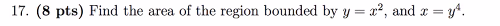### Create an Account

Home / Questions / 17. (8 pts) Find the area of the region bounded by y=x?, and x = y.

# 17. (8 pts) Find the area of the region bounded by y=x?, and x = y.

17. (8 pts) Find the area of the region bounded by y=x?, and x = y.May 05 2021 View more View LessSubscribe To Get Solution